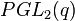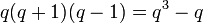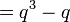# Difference between revisions of "Linear representation theory of projective general linear group of degree two over a finite field"

## Contents

This article gives specific information, namely, linear representation theory, about a family of groups, namely: projective general linear group of degree two.
View linear representation theory of group families | View other specific information about projective general linear group of degree two

This article describes the linear representation theory of the projective general linear group of degree two over a finite field. The order (size) of the field is$q$, and the characteristic prime is$p$.$q$ is a power of$p$. The group is denoted$PGL(2,q)$ or$PGL_2(q)$.

See also the linear representation theory for: special linear group, projective special linear group, and general linear group.

## Summary

Item Value
degrees of irreducible representations over a splitting field Case$q$ odd: 1 (2 times),$q - 1$ ($(q - 1)/2$ times),$q$ (2 times),$q + 1$ ($(q - 3)/2$ times)
Case$q$ even: 1 (1 time),$q - 1$ ($q/2$ times),$q$ (1 time),$q + 1$ ($(q - 2)/2$ times)
number of irreducible representations Case$q$ odd:$q + 2$, case$q$ even:$q + 1$
See number of irreducible representations equals number of conjugacy classes, element structure of projective general linear group of degree two over a finite field#Conjugacy class structure
quasirandom degree (minimum degree of nontrivial ireducible representation) 1
maximum degree of irreducible representation$q + 1$
lcm of degrees of irreducible representations Case$q$ odd:$q(q + 1)(q - 1)/2 = (q^3 - q)/2$; Case$q$ even:$q(q+1)(q-1) = q^3 - q$
sum of squares of degrees of irreducible representations$q(q + 1)(q - 1) = q^3 - q$, equal to the group order; see sum of squares of degrees of irreducible representations equals group order

## Particular cases$q$ (field size)$p$ (underlying prime, field characteristic) Case for$q$ Group$PGL(2,q)$ Order of the group ($= q^3 - q$) Degrees of irreducible representations (ascending order) Number of irreducible representations ($= q + 1$ if$q$ even,$q + 2$ if$q$ odd) Linear representation theory page
2 2 even symmetric group:S3 6 1,1,2 3 linear representation theory of symmetric group:S3
3 3 odd symmetric group:S4 24 1,1,2,3,3 5 linear representation theory of symmetric group:S4
4 2 even alternating group:A5 60 1,3,3,4,5 5 linear representation theory of alternating group:A5
5 5 odd symmetric group:S5 120 1,1,4,4,5,5,6 7 linear representation theory of symmetric group:S5
7 7 odd projective general linear group:PGL(2,7) 336 1,1,6,6,6,7,7,8,8 9 linear representation theory of projective general linear group:PGL(2,7)
8 2 even projective special linear group:PSL(2,8) 504 1,7,7,7,7,8,9,9,9 9 linear representation theory of projective special linear group:PSL(2,8)
9 3 odd projective general linear group:PGL(2,9) 720 1,1,8,8,8,8,9,9,10,10,10 11 linear representation theory of projective general linear group:PGL(2,9)

## Irreducible representations

### Case$p \ne 2$,$q$ odd

Description of collection of representations Parameter for describing each representation How the representation is described Degree of each representation Number of representations Sum of squares of degrees
Trivial --$x \mapsto 1$ 1 1 1
Sign representation -- Kernel is projective special linear group of degree two, image is$\{ \pm 1 \}$ 1 1 1
Unclear a nontrivial homomorphism$\varphi:\mathbb{F}_{q^2}^\ast \to \mathbb{C}^\ast$, with the property that$\varphi(x)^{q + 1} = 1$ for all$x$, and$\varphi$ takes values other than$\pm 1$. Identify$\varphi$ and$\varphi^q$. unclear$q - 1$$(q - 1)/2$$(q - 1)^3/2 = (q^3 - 3q^2 + 3q - 1)/2$
Nontrivial component of permutation representation of$PGL_2$ on the projective line over$\mathbb{F}_q$ -- --$q$ 1$q^2$
Tensor product of sign representation and nontrivial component of permutation representation on projective line -- --$q$ 1$q^2$
Induced from one-dimensional representation of Borel subgroup$\alpha$ homomorphism$\mathbb{F}_q^\ast \to \mathbb{C}^\ast$, with$\alpha$ taking values other than$\pm 1$, up to inverses. Induced from the following representation of the image of the Borel subgroup:$\begin{pmatrix} a & b \\ 0 & d \\\end{pmatrix} \mapsto \alpha(a)\alpha(d)^{-1}$$q + 1$$(q - 3)/2$$(q + 1)^2(q - 3)/2 = (q^3 - q^2 -5q - 3)/2$
Total NA NA NA$q + 2$$q^3 - q$